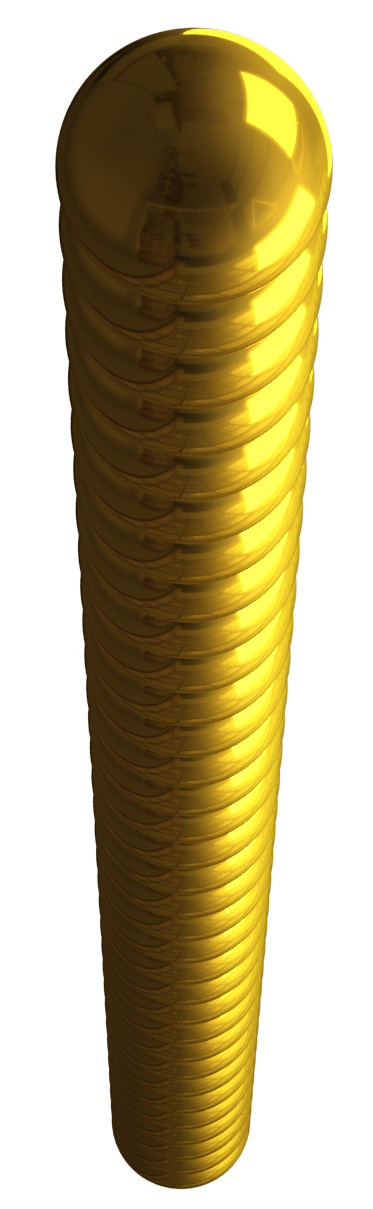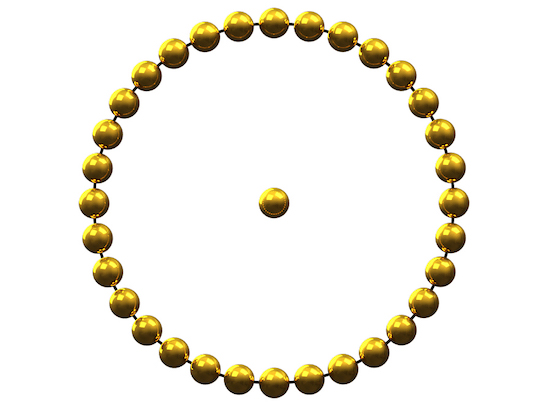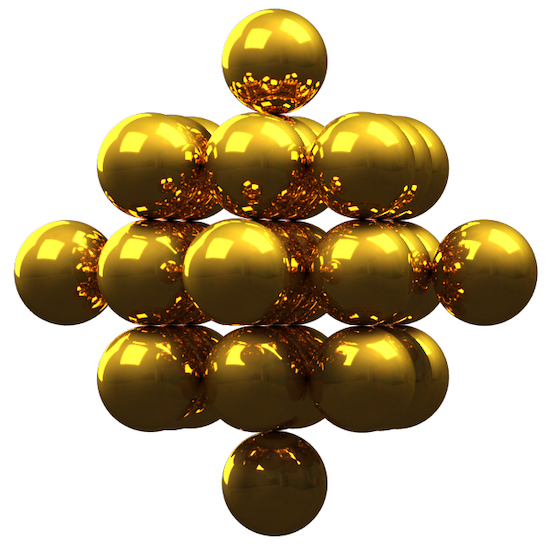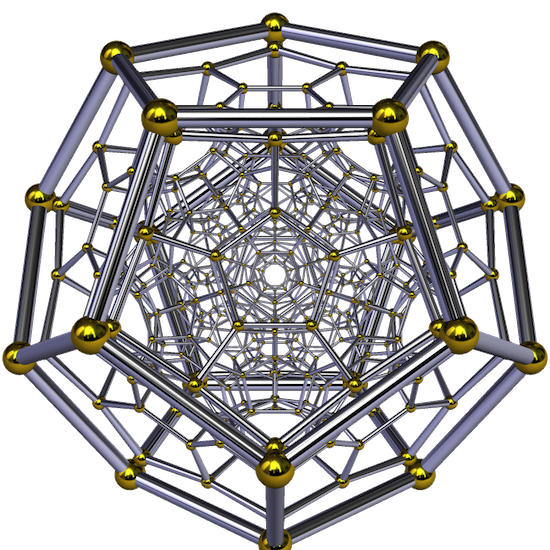# Legend 33

The point (0D) implies the pure potentiality of life itself:The line (1D) implies the 33 vertebrae of the human spine:A centred dodecahedral number (2D) is a centred figurate number that represents trust. The figure below has 33 balls and reminds us of the alchemical symbol for gold:In geometry, the (3D) rhombic dodecahedron is a convex polyhedron with 12 congruent rhombic faces. The rhombic dodecahedron can be used to tessellate 3D space—it can be stacked to fill a space much like hexagons fill a plane. A rhombic dodecahedron’s centre-face-pyramids can be inverted to form a cube. It can also be seen as the 33 balls of faith:In geometry, a hyperdodecahedron is composed of 120 dodecahedral cells with four meeting at each vertex. It is the 4D analogue of the regular dodecahedron, since just as a dodecahedron has 12 pentagonal facets, with three around each vertex, the dodecaplex has 120 dodecahedral facets, with three around each edge. The hyperdodecahedron numbers are 4D figurate numbers based on the 120-cell regular polytope. Starting at a(0) = 33 we find truth:This hyperdodecahedron incorporates the geometries of every convex regular polytope in the first four dimensions. Conversely, it can also be understood by first working with each of its predecessors and the sequence of increasingly complex symmetries they exhibit. So the 120-cell is like a 4D Swiss Army Knife—it contains examples of every relationship among all the convex polytopes found in the first four dimensions.

The unit-radius 120-cell has edge length 1/φ2√2 ≈ 0.270 which can be seen in the animated ratios of our solar system. When working with a number that embodies the universe itself, 33 is a good place to start because it represents life across all dimensions.

You, yourself, as much as anybody in the entire universe, deserve your love and affection.Buddha